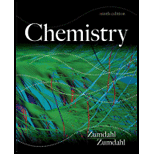# Consider the following reaction: 4 Al ( s ) + 3 O 2 ( g ) → 2 A1 2 O 3 ( s ) It takes 2.00 L of pure oxygen gas at STP to react completely with a certain sample of aluminum. What is the mass of aluminum reacted?### Chemistry

9th Edition
Steven S. Zumdahl
Publisher: Cengage Learning
ISBN: 9781133611097

#### Solutions

Chapter
Section### Chemistry

9th Edition
Steven S. Zumdahl
Publisher: Cengage Learning
ISBN: 9781133611097
Chapter 5, Problem 65E
Textbook Problem
557 views

## Consider the following reaction: 4 Al ( s )   +   3 O 2 ( g ) →   2 A1 2 O 3 ( s ) It takes 2.00 L of pure oxygen gas at STP to react completely with a certain sample of aluminum. What is the mass of aluminum reacted?

Interpretation Introduction

Interpretation:

The mass of aluminum reacted is to be calculated from the given reaction of 4 moles of aluminum and 3 moles of oxygen where 2 L oxygen gas is reacted completely.

Concept introduction:

• STP: standard temperature and pressure, it is the condition of 0oC and 1 atm.

The molar volume of gases at STP condition is similar and it is close to the molar volume of an ideal gas at STP condition. That is, 22.42 L. Hence, at STP the molar volume of a gas is considered as 22.42L.

• Mole ratios between the reactants of a reaction are depends upon the coefficients of respective reactant in a balanced chemical equation.
• Reacted mass of a reactant is the product of number of moles and molecular mass of the reactant.

Givenmass=Number of moles×Molecularmass

To determine: the mass of aluminum reacted in the given reaction of 4 moles of aluminum and 3 moles of oxygen where 2 L oxygen gas is reacted completely.

### Explanation of Solution

Explanation

The given volume of oxygen is 2 L at STP. The molar volume of gases at STP condition is 22.42 L.

So the number of moles of 2 L oxygen at STP is,

Number of molesofO2 =2L×1mole22.42L =0.0892mole

Hence, the number of moles of oxygen reacted is 0.0892 mole

The balance equation of given reaction is 4Al+3O22Al2O3 , here 4 mole of aluminum is needed for 3 moles of oxygen to complete the given reaction. So 4/3 mole of aluminum is reacted for 1 mole of oxygen in the given reaction.

The number of moles of oxygen reacted in the given reaction is calculated from the given volume of 2L at STP as 0.0892 mole. The molar ratio of aluminum in the given reaction is 43 moles of aluminum for 1 mole of oxygen.

Therefore, the number of number of moles of aluminum is needed for given reaction is,

Number of molesofAl =43×0

### Still sussing out bartleby?

Check out a sample textbook solution.

See a sample solution

#### The Solution to Your Study Problems

Bartleby provides explanations to thousands of textbook problems written by our experts, many with advanced degrees!

Get Started

Find more solutions based on key concepts
Moderate alcohol use has been credited with providing possible health benefits. Construct an argument for why m...

Nutrition: Concepts and Controversies - Standalone book (MindTap Course List)

An experiment is performed on a puck on a level air hockey table, where friction is negligible. A constant hori...

Physics for Scientists and Engineers, Technology Update (No access codes included)

Where would you look for water in our solar system?

Oceanography: An Invitation To Marine Science, Loose-leaf Versin